# The notebook

After rising by 40%, the notebook cost 10.50 euros. How much did this notebook cost if it increased in price by only 20% instead of 40%?

x =  9 Eur

### Step-by-step explanation: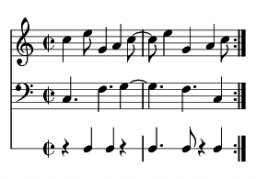Did you find an error or inaccuracy? Feel free to write us. Thank you!Tips to related online calculators

## Related math problems and questions:

• Rising priceThe book first become more expensive by 5 euros. New price was later increased by 8%. After the second price increase book cost 46 euros. What was the original price of the book?
• Price saleoffShoes standing y euros. At first, they were discounted by 12% and then 50% of the new amount. After this double cheapening the cost was exactly 22 euros. Determine the original price of the shoes.
• ChocolateChocolate, which originally cost 1.5 euros, was increased by 40%. How many euros did it cost?
• Discount priceCoat cost 150 euros after sales discount. What is the original price when the discount is 25% of the original price?
• Former priceThe price of an article is cut by 10%, to restore it to the former value, by what percent the new price must be increased?
• Sales off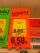shoes cost x euros. First they are discounted by 50%, and then 25% of the new amount. Determine what percentage of the total cost is cheaper.
• Washing machineThe price of washing machine was decreased by 21% and then by 55 € due small sales. After two price decreases cost € 343. How much was originally cost?
• Double price reduction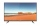The price of TV has been reduced twice. First by 15% and later by another 10% of the reduced price. After this double price reduction, the TV was sold for 8,874 crowns. What was the original price of the TV?
• Ice skates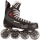Ice skates were raised twice, the first time by 25%, the second time by 10%. After the second price, their cost was 82.5 euros. What was the original price of skates?
• Cost reductionTwo MP3 players whose price was equal to originally have been discounted the first by 20%, the second by 35%. The difference in their prices 750 CZK was after the price reduction. What was the original price of each of the two players?
• The priceThe price of the land increased by 17%. What was the original price of the land if it now costs 46800 €?
• Final priceThe book with the original price of CZK 850 was reduced by 20%. In a week, it was raised by 20% of the new price. Find the final price of the book. How much percents of the original price of the book is the final price?
• Savings and retirement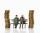The older sibling has saved 44% more euros than the youngest, which represents 22 euros. How many euros older brother has saved ?
• Bike cost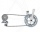The father gave his son € 100 to buy a bicycle, which was 40% of the total amount of the bicycle. How much did the bike cost?
• SkisSkis, which cost € 800, became 25% more expensive before the season. After the season, they became cheaper again by 25%. How many euros did the skis cost after the discount?
• Two shopsIn two different shops, the same skis had the same price. In the first, however, they first became more expensive by 20% and then cheaper by 5%. In the second, they were first cheaper by 5% and then more expensive by 30%, so after these adjustments in the
• Product V2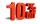The price of product was increased by 12%. Price increased by 273. How much money it would cost this product, if it was on the contrary by 19% discounted.